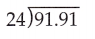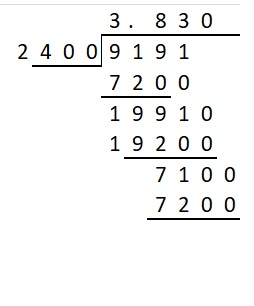# McGraw Hill Math Grade 8 Lesson 9.2 Answer Key Dividing with Decimals

Practice the questions of McGraw Hill Math Grade 8 Answer Key PDF Lesson 9.2 Dividing with Decimals to secure good marks & knowledge in the exams.

## McGraw-Hill Math Grade 8 Answer Key Lesson 9.2 Dividing with Decimals

Exercises

DIVIDE

Question 1.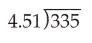74.279
Explanation:
Multiply both the numbers by 100
4.51 x 100 = 451
335 x 100 = 33500
line up a decimal point in the quotient with the decimal point in the divisor.Question 2.58.592
Explanation:
Multiply both the numbers by 10
7.1 x 10 = 71
416 x 10 = 4160
line up a decimal point in the quotient with the decimal point in the divisor.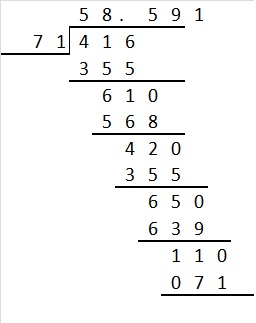Question 3.27.756
Explanation:
Multiply both the numbers by 100
7.71 x 100 = 771
214 x 100 = 21400
line up a decimal point in the quotient with the decimal point in the divisor.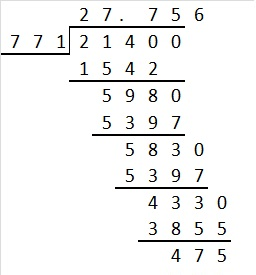Question 4.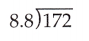19.545
Explanation:
Multiply both the numbers by 10
8.8 x 10 = 88
172 x 10 = 1720
line up a decimal point in the quotient with the decimal point in the divisor.Question 5.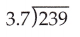64.595
Explanation:
Multiply both the numbers by 10
3.7 x 10 = 37
239 x 10 = 2390
line up a decimal point in the quotient with the decimal point in the divisor.Question 6.7.332
Explanation:
Multiply both the numbers by 100
6.11 x 100 = 611
44.8 x 100 = 4480
line up a decimal point in the quotient with the decimal point in the divisor.Question 7.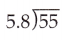9.483
Explanation:
Multiply both the numbers by 10
5.8 x 10 = 58
55 x 10 = 550
line up a decimal point in the quotient with the decimal point in the divisor.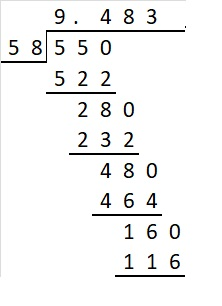Question 8.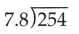32.564
Explanation:
Multiply both the numbers by 10
7.8 x 10 = 78
254 x 10 = 2540
line up a decimal point in the quotient with the decimal point in the divisor.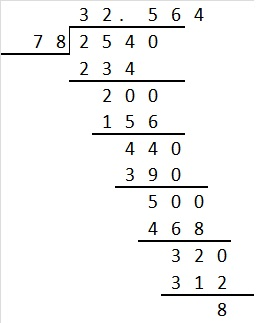Question 9.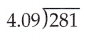68.704
Explanation:
Multiply both the numbers by 100
4.09 x 100 = 409
281 x 100 = 28100
line up a decimal point in the quotient with the decimal point in the divisor.Question 10.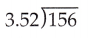44.318
Explanation:
Multiply both the numbers by 100
3.52 x 100 = 352
156 x 100 = 15600
line up a decimal point in the quotient with the decimal point in the divisor.Question 11.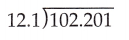8.446
Explanation:
Multiply both the numbers by 1000
12.1 x 1000 = 12100
102.201 x 1000 = 102201
line up a decimal point in the quotient with the decimal point in the dividend.Question 12.